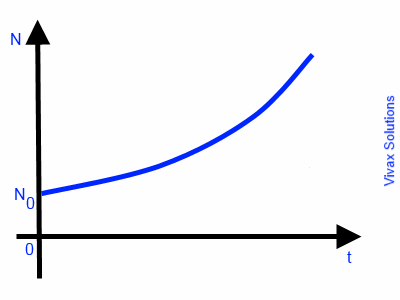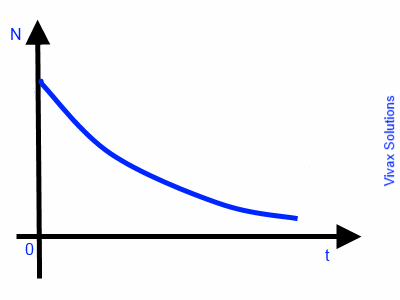# Mathematics

### Differential Equations

An equation in the form of dy/dx = f(x) g(y) is called a differential equation of the first order, where f(x) and g(y) are functions of x and y respectively

E.g.

dy/dx = xy is a differential equation where x and y are functions of x and y respectively.

In order to integrate a differential equation, the variables must be separated as follows:

dy/dx = f(x) g(y)
dy/g(y) = f(x) dx

E.g.1

dy/dx = xy
∫ dy/y = ∫ x dx
ln y = x2/2 + c
y = ex2 + c

E.g.2

dy/dx = cos2y ex
dy/cos2y = ex dx
∫ dy/cos2y = ∫ ex dx
∫ dy sec2y = ∫ ex dx
tan y = ex + c

#### Exponential Growth / Decay

If a rate of change is proportional to its quantity, such a rate is called Exponential Growth / Decay.

E.g.1

The rate of increase in population of a colony of bacteria is proportional to the number of bacteria in the colony at a given time. Therefore, such a rate of increase is an exponential growth.

So, dN/dt ∝ N
dN/dt = k N
dN/N = k dt
∫ dN/N = ∫ k dt
ln N = kt + c
N = ekt + c
N = ekt X ec ---- 1
Let N = N0 when t = 0
N0 = ec
Sub this in 1
N = N0ekt
This is exponential growth. The following image indicates the graphical nature of the growth.E.g.2

The rate of decay of radioactive nuclei in a radioactive substance is directly proportional to the number of remaining nuclei at a given time. Therefore, this is an exponential decay.

So, dN/dt ∝ -N ---- the negative sign indicates a decay or a loss
dN/dt = -k N
dN/N = -k dt
∫ dN/N = ∫ k dt
ln N = -kt + c
N = e-kt + c
N = e-kt X ec ---- 1
Let N = N0 when t = 0
N0 = ec
Sub this in 1
N = N0e-kt
This is exponential decay. The following image indicates the graphical nature of the decay against time.Maths is challenging; so is finding the right book. K A Stroud, in this book, cleverly managed to make all the major topics crystal clear with plenty of examples; popularity of the book speak for itself - 7th edition in print.

### Recommended - GCSE & iGCSEThis is the best book available for the new GCSE(9-1) specification and iGCSE: there are plenty of worked examples; a really good collection of problems for practising; every single topic is adequately covered; the topics are organized in a logical order.

### Recommended for A LevelThis is the best book that can be recommended for the new A Level - Edexcel board: it covers every single topic in detail;lots of worked examples; ample problems for practising; beautifully and clearly presented.